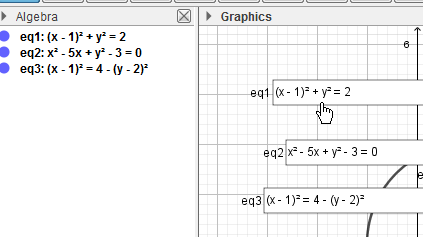# conic equation in input form

rsingkhuwal4445 shared this question 2 years ago

How can i fix the equation of circle in input form without changing into input form everytime I change the equation from input box i get it in standard form.1

change the form of eq to input form before creating the inputbox1

sorry to mention but it didn't work.1

Also it will probably work better if you set the Input Box to symbolic (Algebra -> Symbolic)1

sorry to mention but it didn't work.11

The file is attached here.1

perhaps I do not understaND YOU1

I created input box after changing equation i to input form but when i change equation in input box, the output is always in (x-a)^2+(y-b)^2=r^2.1

now I see

I think there is possible bug1

What bug?1

OK, I see1

even online is showing same problem1

si la conica es una elipse se ve en forma general correctamente pero no consigo que el input_form se mantenga como formato de salida